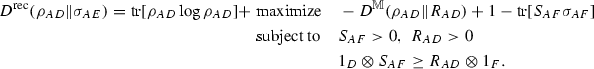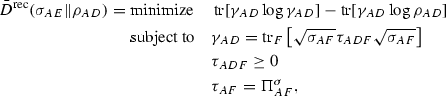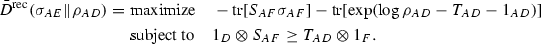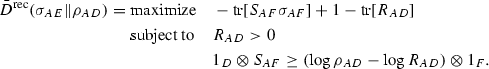# On variational expressions for quantum relative entropies

## Abstract

Distance measures between quantum states like the trace distance and the fidelity can naturally be defined by optimizing a classical distance measure over all measurement statistics that can be obtained from the respective quantum states. In contrast, Petz showed that the measured relative entropy, defined as a maximization of the Kullback–Leibler divergence over projective measurement statistics, is strictly smaller than Umegaki’s quantum relative entropy whenever the states do not commute. We extend this result in two ways. First, we show that Petz’ conclusion remains true if we allow general positive operator-valued measures. Second, we extend the result to Rényi relative entropies and show that for non-commuting states the sandwiched Rényi relative entropy is strictly larger than the measured Rényi relative entropy for $$\alpha \in (\frac{1}{2}, \infty )$$ and strictly smaller for $$\alpha \in [0,\frac{1}{2})$$. The latter statement provides counterexamples for the data processing inequality of the sandwiched Rényi relative entropy for $$\alpha < \frac{1}{2}$$. Our main tool is a new variational expression for the measured Rényi relative entropy, which we further exploit to show that certain lower bounds on quantum conditional mutual information are superadditive.

This is a preview of subscription content, access via your institution.

1. 1.

P and Q are orthogonal, denoted $$P \perp Q$$, if there exists an $$A \subseteq \mathcal {X}$$ such that $$P(A) = 1$$ and $$Q(A) = 0$$.

2. 2.

A very recent preprint  has demonstrated by numerical examples that the relative entropy of recovery is indeed non-additive.

## References

1. 1.

Alberti, P.M.: A note on the transition probability over C*-algebras. Lett. Math. Phys. 7, 25–32 (1983)

2. 2.

Araki, H.: On an inequality of lieb and thirring. Lett. Math. Phys. 19(2), 167–170 (1990)

3. 3.

Beigi, S.: Sandwiched Rényi divergence satisfies data processing inequality. J. Math. Phys. 54(12), 122202 (2013)

4. 4.

Belavkin, V.P., Staszewski, P.: C*-algebraic generalization of relative entropy and entropy. Ann. Henri Poincaré 37(1), 51–58 (1982)

5. 5.

Ben-Tal, A., Nemirovski, A.: Lectures on Modern Convex Optimization. Society for Industrial and Applied Mathematics, Philadelphia (2001)

6. 6.

Berta, M., Tomamichel, M.: The fidelity of recovery is multiplicative. IEEE Trans. Inf. Theory 62(4), 1758–1763 (2016)

7. 7.

Boyd, S., Vandenberghe, L.: Convex Optimization. Cambridge University Press, Cambridge (2004)

8. 8.

Brandão, F.G.S.L., Harrow, A.W., Oppenheim, J., Strelchuk, S.: Quantum conditional mutual information, reconstructed states, and state redistribution. Phys. Rev. Lett. 115(5), 050501 (2015)

9. 9.

Chandrasekaran, V., Shah, P.: Relative entropy optimization and its applications. Math. Program. 161(1), 1–32 (2017)

10. 10.

DiVincenzo, D., Horodecki, M., Leung, D., Smolin, J., Terhal, B.: Locking classical correlations in quantum states. Phys. Rev. Lett. 92(6), 067902 (2004)

11. 11.

Donald, M.J.: On the relative entropy. Commun. Math. Phys. 105(1), 13–34 (1986)

12. 12.

Farenick, D.R.: Private Communication (2015)

13. 13.

Farenick, D.R., Zhou, F.: Jensen’s inequality relative to matrix-valued measures. J. Math. Anal. Appl. 327(2), 919–929 (2007)

14. 14.

Fawzi, H., Fawzi, O.: Relative entropy optimization in quantum information theory via semidefinite programming approximations (2017). arXiv:1705.06671 (submitted)

15. 15.

Fawzi, O., Renner, R.: Quantum conditional mutual information and approximate Markov chains. Commun. Math. Phys. 340(2), 575–611 (2015)

16. 16.

Frank, R.L., Lieb, E.H.: Monotonicity of a relative Rényi entropy. J. Math. Phys. 54(12), 122201 (2013)

17. 17.

Fuchs, C.A.: Distinguishability and accessible information in quantum theory. Ph.D. Thesis, University of New Mexico (1996). arXiv:quant-ph/9601020v1

18. 18.

Golden, S.: Lower bounds for the Helmholtz function. Phys. Rev. 137(4B), B1127–B1128 (1965)

19. 19.

Hiai, F.: Equality cases in matrix norm inequalities of Golden-Thompson type. Linear Multilinear Algebra 36(4), 239–249 (1994)

20. 20.

Hiai, F., Petz, D.: The proper formula for relative entropy and its asymptotics in quantum probability. Commun. Math. Phys. 143(1), 99–114 (1991)

21. 21.

Cooney, T., Hirche, C., Morgan, C., Olson, J.P., Seshadreesan, K.P., Watrous, J., Wilde, M.M.: Operational meaning of quantum measures of recovery. Phys. Rev. A. 94(2), 022310 (2016)

22. 22.

Ibinson, B., Linden, N., Winter, A.: Robustness of quantum Markov chains. Commun. Math. Phys. 277(2), 289–304 (2007)

23. 23.

Junge, M., Renner, R., Sutter, D., Wilde, M.M., Winter, A.: Universal recovery from a decrease of quantum relative entropy (2015). arXiv:1509.07127 (Preprint)

24. 24.

König, R., Renner, R., Schaffner, C.: The operational meaning of min- and max-entropy. IEEE Trans. Inf. Theory 55(9), 4337–4347 (2009)

25. 25.

Kosaki, H.: Relative entropy of states: a variational expression. J. Oper. Theory 16(2), 335–348 (1986)

26. 26.

Kullback, S., Leibler, R.A.: On information and sufficiency. Ann. Math. Stat. 22(1), 79–86 (1951)

27. 27.

Li, K., Winter, A.: Squashed entanglement, k-extendibility, quantum markov chains, and recovery maps (2014). arXiv:1410.4184 (Preprint)

28. 28.

Lieb, E.H., Thirring, W.E.: Inequalities for the moments of the eigenvalues of the Schrödinger Hamiltonian and their relation to Sobolev inequalities. In: Thirring, W. (ed) The Stability of Matter: From Atoms to Stars, chapter III, pp. 205–239. Springer, Berlin (2005)

29. 29.

Lindblad, G.: Completely positive maps and entropy inequalities. Commun. Math. Phys. 40(2), 147–151 (1975)

30. 30.

Matsumoto, K.: A new quantum version of f-divergence (2014). arXiv:1311.4722 (Preprint)

31. 31.

Mosonyi, M., Ogawa, T.: Quantum hypothesis testing and the operational interpretation of the quantum Rényi relative entropies. Commun. Math. Phys. 334(3), 1617–1648 (2015)

32. 32.

Müller-Lennert, M.: Quantum relative Rényi entropies. Master Thesis, ETH Zurich

33. 33.

Müller-Lennert, M., Dupuis, F., Szehr, O., Fehr, S., Tomamichel, M.: On quantum Rényi entropies: a new generalization and some properties. J. Math. Phys. 54(12), 122203 (2013)

34. 34.

Petz, D.: Quasi-entropies for finite quantum systems. Rep. Math. Phys. 23(1), 57–65 (1986)

35. 35.

Petz, D.: Sufficient subalgebras and the relative entropy of states of a von Neumann algebra. Commun. Math. Phys. 105(1), 123–131 (1986)

36. 36.

Petz, D.: A variational expression for the relative entropy. Commun. Math. Phys. 114(2), 345–349 (1988)

37. 37.

Petz, D.: Quantum Information Theory and Quantum Statistics. Springer, Berlin (2008)

38. 38.

Piani, M.: Relative entropy of entanglement and restricted measurements. Phys. Rev. Lett. 103(16), 160504 (2009)

39. 39.

Rains, E.: A semidefinite program for distillable entanglement. IEEE Trans. Inf. Theory 47(7), 2921–2933 (2001)

40. 40.

Rényi, A.: On measures of information and entropy. In: Proceedings of 4th Berkeley Symposium on Mathematical Statistics and Probability, vol. 1, pp. 547–561. Berkeley, California, USA (1961)

41. 41.

Seshadreesan, K.P., Wilde, M.M.: Fidelity of recovery, squashed entanglement, and measurement recoverability. Phys. Rev. A 92(4), 042321 (2015)

42. 42.

Sion, M.: On general minimax theorems. Pac. J. Math. 8, 171–176 (1958)

43. 43.

So, W.: Equality cases in matrix exponential inequalities. SIAM J. Matrix Anal. Appl. 13(4), 1154–1158 (1992)

44. 44.

Sutter, D., Fawzi, O., Renner, R.: Universal recovery map for approximate Markov chains. Proc. R. Soc. Lond. A Math. Phys. Eng. Sci. 472, 2186 (2016)

45. 45.

Sutter, D., Tomamichel, M., Harrow, A.W.: Strengthened monotonicity of relative entropy via pinched Petz recovery map. IEEE Trans. Inf. Theory 62(5), 2907–2913 (2016)

46. 46.

Thompson, C.J.: Inequality with applications in statistical mechanics. J. Math. Phys. 6(11), 1812 (1965)

47. 47.

Tomamichel, M.: Quantum Information Processing with Finite Resources-Mathematical Foundations, volume 5 of SpringerBriefs in Mathematical Physics. Springer, Berlin (2016)

48. 48.

Tomamichel, M., Berta, M., Hayashi, M.: Relating different quantum generalizations of the conditional Rényi entropy. J. Math. Phys. 55(8), 082206 (2014)

49. 49.

Uhlmann, A.: Relative entropy and the Wigner-Yanase-Dyson-Lieb concavity in an interpolation theory. Commun. Math. Phys. 54(1), 21–32 (1977)

50. 50.

Umegaki, H.: Conditional expectation in an operator algebra. Kodai Math. Sem. Rep. 14, 59–85 (1962)

51. 51.

van Erven, T., Harremoes, P.: Rényi divergence and Kullback-Leibler divergence. IEEE Trans. Inf. Theory 60(7), 3797–3820 (2014)

52. 52.

Vedral, V.: On bound entanglement assisted distillation. Phys. Lett. A 262(2–3), 121–124 (1999)

53. 53.

Vedral, V., Plenio, M.B.: Entanglement measures and purification procedures. Phys. Rev. A 57(3), 1619–1633 (1998)

54. 54.

Wilde, M.M.: Recoverability in quantum information theory. Proc. R. Soc. A 471(2182), 20150338 (2015)

55. 55.

Wilde, M.M., Winter, A., Yang, D.: Strong converse for the classical capacity of entanglement-breaking and Hadamard channels via a sandwiched Rényi relative entropy. Commun. Math. Phys. 331(2), 593–622 (2014)

Download references

## Acknowledgements

We acknowledge discussions with Fernando Brandão, Douglas Farenick and Hamza Fawzi. MB acknowledges funding by the SNSF through a fellowship, funding by the Institute for Quantum Information and Matter (IQIM), an NSF Physics Frontiers Center (NFS Grant PHY-1125565) with support of the Gordon and Betty Moore Foundation (GBMF-12500028), and funding support form the ARO grant for Research on Quantum Algorithms at the IQIM (W911NF-12-1-0521). Most of this work was done while OF was also with the Department of Computing and Mathematical Sciences, California Institute of Technology. MT would like to thank the IQIM at CalTech and John Preskill for his hospitality during the time most of the technical aspects of this project were completed. He is funded by an ARC Discovery Early Career Researcher Award fellowship (Grant No. DE160100821).

## Author information

Authors

### Corresponding author

Correspondence to Mario Berta.

## Appendix A: Proofs for results in Sect. 5

### Proof of Lemma 10

We start by writing out the relative entropy of recovery as a convex optimization program,(A1)

where the notation is as in the proof of Lemma 8. Clearly, the first and last constraint can be relaxed to $$\gamma _{AD} \le {{\mathrm{tr}}}_F\left[ \sqrt{\sigma _{AF}}\tau _{ADF}\sqrt{\sigma _{AF}}\right]$$ and $$\tau _{AF} \le \Pi ^\sigma _{AF}$$ without changing the solution. The Lagrangian can then be written as

\begin{aligned} \mathcal {L}(\gamma , \tau , R, S)&= - {{\mathrm{tr}}}[\rho _{AD} \log \gamma _{AD}]+{{\mathrm{tr}}}\left[ R_{AD}\left( \gamma _{AD}-{{\mathrm{tr}}}_F\left[ \sqrt{\sigma _{AF}}\tau _{ADF}\sqrt{\sigma _{AF}}\right] \right) \right] \nonumber \\&\quad +{{\mathrm{tr}}}\left[ S_{AF}\left( \tau _{AF}-\Pi ^\sigma _{AF}\right) \right] \end{aligned}
(A2)
\begin{aligned}&= - {{\mathrm{tr}}}[\rho _{AD} \log \gamma _{AD}]+{{\mathrm{tr}}}[R_{AD}\gamma _{AD}]\nonumber \\&\quad -{{\mathrm{tr}}}\left[ \tau _{ADF}\left( \sqrt{\sigma _{AF}}R_{AD}\sqrt{\sigma _{AF}}-S_{AF}\right) \right] \nonumber \\&\quad -{{\mathrm{tr}}}\left[ S_{AF}\Pi ^\sigma _{AF}\right] \, , \end{aligned}
(A3)

where we introduced the variables $$R_{AD} \ge 0$$ and $$S_{AF} \ge 0$$. In order to compute the dual objective function, we should take the infimum of this quantity over $$\gamma _{AD}>0$$ and $$\tau _{ADF} \ge 0$$. Using the variational expression for the measured relative entropy (Lemma 1), we find

\begin{aligned} \inf _{\gamma _{AD}>0,\,\tau _{ADF} \ge 0} \mathcal {L}(\gamma , \theta , R, S) =-D^{\mathbb {M}}(\rho _{AD}\Vert R_{AD})+1-{{\mathrm{tr}}}[S_{AF}\Pi ^\sigma _{AF}], \end{aligned}
(A4)

when $$S_{AF}\ge \sqrt{\sigma _{AF}}R_{AD}\sqrt{\sigma _{AF}}$$. With Slater’s strong duality and a change of variable $$\bar{S}_{AF}:=\sigma _{AF}^{-1/2}S_{AF}\sigma _{AF}^{-1/2}$$ (but not including the bar in the following), we get(A5)

Adding the constraint $${{\mathrm{tr}}}[S_{AF}\sigma _{AF}]=1$$ as in the proof of Lemma 8 concludes the proof. $$\square$$

### Proof of Proposition 11

We start by writing out the argument flipped relative entropy of recovery as a convex optimization program,(A6)

where the notation is as in the proof of Lemma 8. The Lagrangian can be written as

\begin{aligned} \mathcal {L}(\gamma , \tau , T, S)&= {{\mathrm{tr}}}[\gamma _{AD} \log \gamma _{AD}]-{{\mathrm{tr}}}[\gamma _{AD} \log \rho _{AD}]\nonumber \\&\quad +{{\mathrm{tr}}}\left[ T_{AD}\left( \gamma _{AD}-{{\mathrm{tr}}}_F\left[ \sqrt{\sigma _{AF}}\tau _{ADF}\sqrt{\sigma _{AF}}\right] \right) \right] \nonumber \\&\quad +{{\mathrm{tr}}}\left[ S_{AF}\left( \tau _{AF}-\Pi ^\sigma _{AF}\right) \right] \end{aligned}
(A7)
\begin{aligned}&= {{\mathrm{tr}}}[\gamma _{AD} \log \gamma _{AD}]+{{\mathrm{tr}}}[\gamma _{AD}(T_{AD}-\log \rho _{AD})]\nonumber \\&\quad -{{\mathrm{tr}}}\left[ S_{AF}\Pi ^\sigma _{AF}\right] -{{\mathrm{tr}}}\left[ \tau _{ADF}\left( \sqrt{\sigma _{AF}}T_{AD}\sqrt{\sigma _{AF}}-S_{AF}\right) \right] , \end{aligned}
(A8)

where $$T_{AD}$$ and $$S_{AF}$$ are Hermitian operators. In order to compute the dual objective function, we should take the infimum of this quantity over $$\gamma _{AD}>0$$ and $$\tau _{ADF} \ge 0$$. From the last expression we get $$S_{AF}\ge \sqrt{\sigma _{AF}}T_{AD}\sqrt{\sigma _{AF}}$$ and we also know how to optimize the first expression as it is an entropy maximization question:

\begin{aligned} \inf _{\gamma _{AD}>0} {{\mathrm{tr}}}[\gamma _{AD} \log \gamma _{AD}]+{{\mathrm{tr}}}[\gamma _{AD}(T_{AD}-\log \rho _{AD})]. \end{aligned}
(A9)

It is optimized when $$\gamma _{AD} = \exp (\log \rho _{AD} - T_{AD} + \alpha 1_{AD})$$ for some $$\alpha$$ . This means that this infimum is given by

\begin{aligned}&\inf _{\alpha } {{\mathrm{tr}}}[\exp (\log \rho _{AD} - T_{AD} + \alpha 1_{AD}) \left( \log \rho _{AD} - T_{AD} + \alpha 1_{AD} \right) ]\nonumber \\&\quad + {{\mathrm{tr}}}[\exp (\log \rho _{AD} - T_{AD} + \alpha 1_{AD}) \left( T_{AD} - \log \rho _{AD} \right) ]\nonumber \\&= \inf _{\alpha } \alpha {{\mathrm{tr}}}[\exp (\log \rho _{AD} - T_{AD} + \alpha 1_{AD})] \end{aligned}
(A10)
\begin{aligned}&= \inf _{\alpha } \alpha e^{\alpha } {{\mathrm{tr}}}[\exp (\log \rho _{AD} - T_{AD})] \end{aligned}
(A11)
\begin{aligned}&= - {{\mathrm{tr}}}[\exp (\log \rho _{AD} -T_{AD} - 1_{AD})]. \end{aligned}
(A12)

With Slater’s strong duality and a change of variable $$\bar{S}_{AF}:=\sigma _{AF}^{-1/2}S_{AF}\sigma _{AF}^{-1/2}$$ (but not including the bar in the following), we get(A13)

We now do another change of variable (but not including the bar in the following)

\begin{aligned} R_{AD}:= \exp (\log \rho _{AD} - T_{AD} - 1_{AD})\quad \text {as well as}\quad 1_{D}\otimes \bar{S}_{AF}:=1_{D}\otimes S_{AF}+1_{ADF}, \end{aligned}
(A14)

and the program becomes(A15)

Observe now that we can add the constraint $${{\mathrm{tr}}}[R_{AD}] = 1$$. In fact, let

\begin{aligned} \bar{R}_{AD}:= \frac{R_{AD}}{{{\mathrm{tr}}}[R_{AD}]}\quad \mathrm {and}\quad \bar{S}_{AF}:= S_{AF} + \log {{\mathrm{tr}}}[R_{AD}]. \end{aligned}
(A16)

This solution satisfies the constraint and the objective value becomes

\begin{aligned} -{{\mathrm{tr}}}[\bar{S}_{AF}\sigma _{AF}]+1- {{\mathrm{tr}}}[\bar{R}_{AD}]&= - {{\mathrm{tr}}}[S_{AF}\sigma _{AF}] - \log {{\mathrm{tr}}}[R_{AD}] \nonumber \\&\ge - {{\mathrm{tr}}}[S_{AF}\sigma _{AF}] + 1 - {{\mathrm{tr}}}[R_{AD}]. \end{aligned}
(A17)

This concludes the proof of (96).

To prove (97), first note that it is immediate from the definition of the argument flipped relative entropy of recovery that

\begin{aligned} \bar{D}^{\mathrm {rec}}(\sigma _{AE}\otimes \omega _{A'E'}\Vert \rho _{AD}\otimes \tau _{A'D'})\le \bar{D}^{\mathrm {rec}}(\sigma _{AE}\Vert \rho _{AD})+\bar{D}^{\mathrm {rec}}(\omega _{A'E'}\Vert \tau _{A'D'}), \end{aligned}
(A18)

and in the following we prove inequality in the other direction using the dual representation (96). Given feasible operators $$R_{AD},S_{AF}$$ for the quantity $$\bar{D}^{\mathrm {rec}}(\sigma _{AE}\Vert \rho _{AD})$$ and feasible operators $$R_{A'D'},S_{A'F'}$$ for the quantity $$\bar{D}^{\mathrm {rec}}(\omega _{A'E'}\Vert \tau _{A'D'})$$, we have

\begin{aligned}&1_D\otimes S_{AF}\ge (\log \rho _{AD}-\log R_{AD})\otimes 1_F \wedge \ 1_{D'}\otimes S_{A'F'}\nonumber \\&\quad \ge (\log \tau _{A'D'}-\log R_{A'D'})\otimes 1_{F'} \nonumber \\&\implies 1_{DD'}\otimes (S_{AF}\otimes 1_{A'F'}+1_{AF}\otimes S_{A'F'})\nonumber \\&\quad \quad \;\;\ge \big ((\log \rho _{AD}-\log R_{AD})\otimes 1_{A'D'}+1_{AD}\otimes (\log \tau _{A'D'}-\log R_{A'D'})\big )\otimes 1_{FF'}\nonumber \\&\quad \quad \;\;=\big (\log (\rho _{AD}\otimes \tau _{A'D'})-\log (R_{AD}\otimes R_{A'D'})\big )\otimes 1_{FF'}, \end{aligned}
(A19)

just by multiplying with identities and adding the resulting operator inequalities. Moreover, we have $${{\mathrm{tr}}}[R_{AD}\otimes R_{A'D'}]=1$$. Hence, $$(R_{AD}\otimes R_{A'D'},S_{AF}\otimes 1_{A'F'}+1_{AF}\otimes S_{A'F'})$$ is a feasible pair in the expression (96) for $$\bar{D}^{\mathrm {rec}}(\sigma _{AE}\otimes \omega _{A'E'}\Vert \rho _{AD}\otimes \tau _{A'D'})$$ and we get

\begin{aligned}&\bar{D}^{\mathrm {rec}}(\sigma _{AE}\otimes \omega _{A'E'}\Vert \rho _{AD}\otimes \tau _{A'D'})\nonumber \\&\quad \ge -{{\mathrm{tr}}}[(S_{AF}\otimes 1_{A'F'}+1_{AF}\otimes S_{A'F'})(\sigma _{AF}\otimes \omega _{A'F'})] \end{aligned}
(A20)
\begin{aligned}&\quad =-{{\mathrm{tr}}}[S_{AF}\sigma _{AF}]-{{\mathrm{tr}}}[S_{A'F'}\omega _{A'F'}]. \end{aligned}
(A21)

Taking the supremum over feasible $$(R_{AD},S_{AF})$$ and $$(R_{A'D'},S_{A'F'})$$, we find the claimed additivity. $$\square$$

## Rights and permissions

Reprints and Permissions